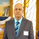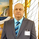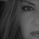19720 lượt xem
Introduced by M.H.Pee, Trend Trigger Factor is designed to keep the trader trading with the trend.

System rules according to the developer:
* If the 15-day TTF is above 100 (indicating an uptrend), you will want to be in long positions.
* If the 15-day TTF is below -100, you will want to be short.
* If it is between -100 and 100, you should remain with the current position.

```//
// @author LazyBear
//
study("Trend Trigger Factor [LazyBear]", shorttitle="TTF_LB")
length=input(15)
bt = input( 100, title="Buy Trigger")
st = input( -100, title="Sell Trigger")
markCrossovers=input(false, type=bool)

prev(s,i) =>
y=abs(round(i))
s[y]

calc_ttf( periods ) =>
bp = highest( high, periods ) - prev( lowest( low, periods ), - periods )
sp = prev( highest( high, periods ), - periods ) - lowest( low, periods )
100 * (bp - sp) / ( 0.5*( bp + sp) )

ttf = calc_ttf( length )
plot(0, color=gray)
btl=plot(bt, color=gray, style=3)
stl=plot(st, color=gray, style=3)

long_f = cross( ttf, st ) and rising(ttf, 1)
short_f = cross(ttf, bt ) and falling(ttf, 1)

bs = (ttf > bt) ? bt : ttf
us = (ttf < st) ? st : ttf
bl=plot(bs, color=white)
ul=plot(us, color=white)
tl=plot(ttf, title="TTF", color=markCrossovers ? (long_f ? green : short_f ? red : blue) : maroon, linewidth=2)
fill(bl, tl, color=green, transp=75)
fill(ul, tl, color=red, transp=75)
```
List of my free indicators: http://bit.ly/1LQaPK8
List of my indicators at Appstore: http://blog.tradingview.com/?p=970

## Bình luậnInteresting in understanding the following formula, for which values of s and i dont understand. prev(s,i) =>
y=abs(round(i))
s Thanks.
Phản hồiwhat kind of statement the following codes are? A switch statement: #prev(s,i) =>
# y=abs(round(i))
# s

#calc_ttf( periods ) =>
# bp = highest( high, periods ) - prev( lowest( low, periods ), - periods )
# sp = prev( highest( high, periods ), - periods ) - lowest( low, periods )
# 100 * (bp - sp) / ( 0.5*( bp + sp) )

Thanks.
Phản hồiYou should be advanced into a Mod position:) You would be the only one that earned it in a hard way. Great and appreciate it work.
Phản hồi